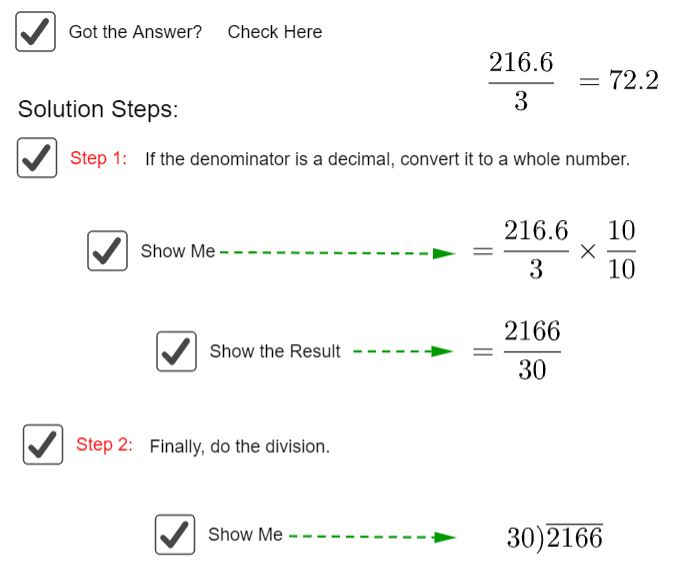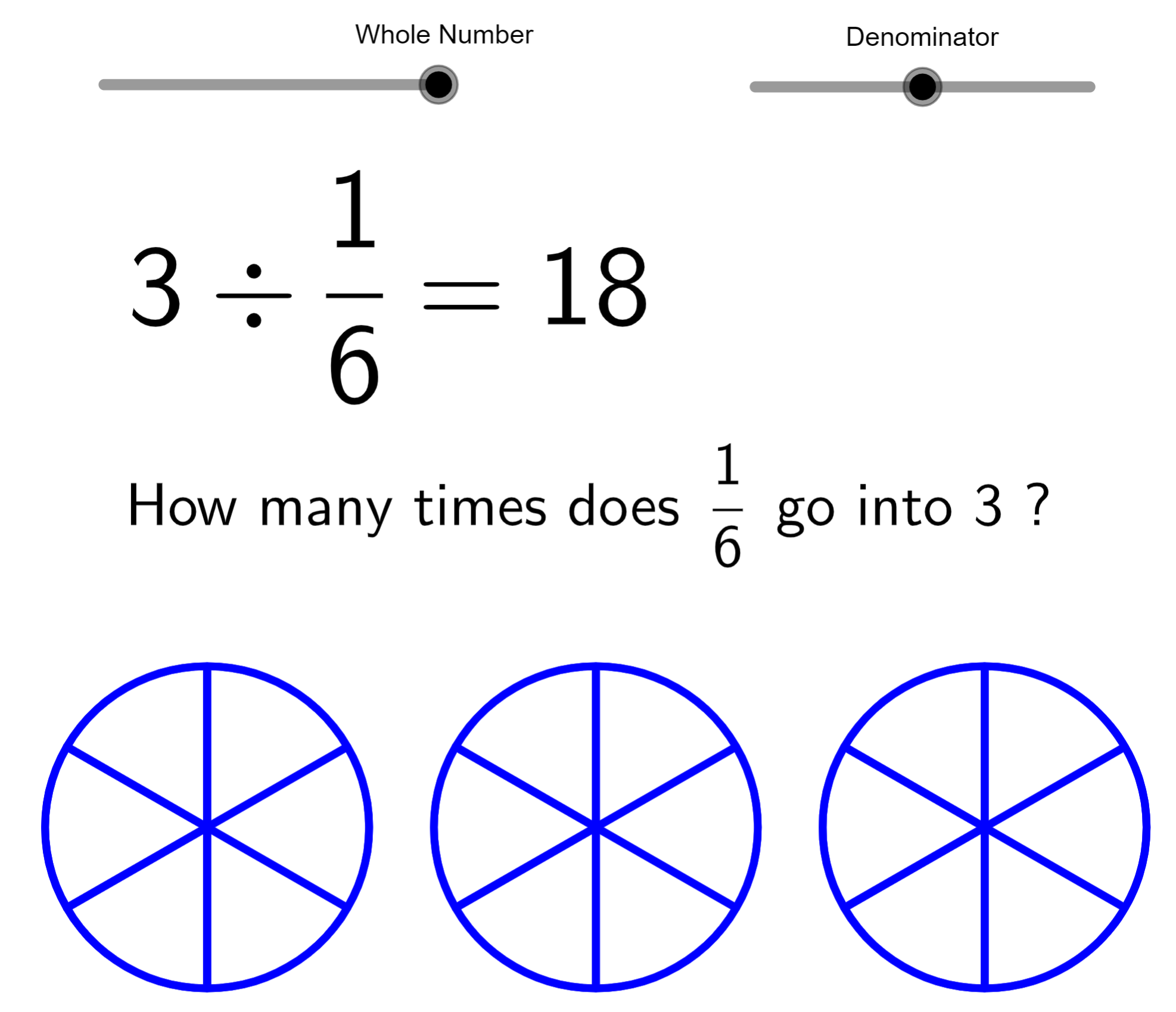# Division of Decimals

Division of Decimals
Go back to  'Decimals'

## Introduction to Division of Decimals

Decimals always get us worked up by themselves. And when we need to use an operation like addition, subtraction, multiplication or division on decimals, they become even scarier. However, the reality is that if the base ten of decimals is understood and decimal numbers are manipulated accordingly then these operations become very simple.

## The Big Idea: What is involved in the division of decimals?

The algorithms built around the division of whole numbers translate perfectly to Division of decimals

When we spoke of division of whole numbers, we introduced the concept of the Remainder. Well, as it turns out, math likes elegant solutions and doesn’t like loose ends, so it devised a way to deal with the remainder in terms of repeated subtractions or equal distribution. That is the core concept behind the division of decimals.

Here, take a look at the steps involved in the division of decimals for yourself!## Why is it important to understand division of decimals?

Decimals impart us with precision. There are multiple scenarios in which one would require and value precision to pinpoint accuracy, such as most mechanical engineering projects or space travel. This necessitates that math develops tools that allows us to make precise calculations and hence we have formulated ways to extend the basic operations to apply to decimals perfectly as well.

### How to Divide Decimals by whole numbers or decimals

When a decimal is to be multiplied by a whole number, the decimal point of both numbers is to be moved as many times to the right as is required to show both numbers as whole numbers. So, if 34.287 is to be divided by 9, then you should consider it as $${34287 \over 9000}$$. Similarly, if 3.427 is to be divided by 0.28 then you should consider it as $${3427 \over 280}$$. If this method is followed, then unlike the multiplication process, there is no further need to move back the decimal point.### How to divide two decimals?

Well, like we said before, the concept of dividing decimals can be directly taken from the division of whole numbers. The same rules apply, including those for long division. Simply keep dividing the two numbers (in case of long division) until the quotient terminates or starts repeating. Here, take a look:### An easy way to divide decimals lesser than 1

The fun thing about dividing two decimal numbers each other when they are both less than 1 is that the division can be done in a much easier way than it looks. Both the numbers can easily be expressed as proper fractions with the denominator expressed as a number with base ten. Let us see how this works, with the help of a small example. Let us see how to divide 0.34 by 0.14 where both numbers are decimals less than 1.

\begin{align} & \frac{{0.34}}{{0.14}}\\ & = \frac{{\left( {\frac{{34}}{{100}}} \right)}}{{\left( {\frac{{14}}{{100}}} \right)}}\\ & = \left( {\frac{{34}}{{100}}} \right) \times \left( {\frac{{100}}{{14}}} \right)\\ & = \frac{{34}}{{14}}\\ & = 2.43 \end{align}

The above division simply took place as a division of 34 by 14, because the 100s cancelled each other out.

More Important Topics
Numbers
Algebra
Geometry
Measurement
Money
Data
Trigonometry
Calculus
More Important Topics
Numbers
Algebra
Geometry
Measurement
Money
Data
Trigonometry
Calculus
Learn from the best math teachers and top your exams

• Live one on one classroom and doubt clearing
• Practice worksheets in and after class for conceptual clarity
• Personalized curriculum to keep up with school